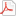# Test Information Guide

## Field 12: Chemistry Sample Multiple-Choice Questions

The following reference material will be available to you during the test:

Formulas/ConstantsPeriodic Table### Nature of Science and Properties of Matter

##### Objective 0002Understand scientific tools, instruments, materials, and safety practices.

1. Which of the following activities is most likely to lead to gas burning in the base of the barrel of a Bunsen burner?

1. using the burner under low gas pressure conditions
2. igniting the burner with a sparker held 5 cm from the top of the burner
3. connecting the source gas to the burner with rubber tubing
4. turning the source gas flow valve to the maximally opened position
Correct Response: A.

Correct Response: A.

##### Objective 0003Understand the relationships among science, technology, and society.

2. The growing perception that carbon dioxide is involved in global climate change has led to which of the following?

1. the removal of lead compounds from gasoline
2. a decrease in the use of catalytic converters
3. the development of chloro-flourocarbon-free refrigerants
4. a renewed interest in wind and solar energy sources
Correct Response: D.

Correct Response: D.

##### Objective 0004Understand chemical and physical properties and changes in matter.

3. Which of the following is a characteristic that distinguishes a solution from a colloid?

1. Solids in a solution can be filtered out of a solution.
2. Solids in a colloid will scatter light.
3. Solids will eventually settle out of a colloid.
4. Solids outweigh the water component in a solution.
Correct Response: B.

Correct Response: B.

### Atomic Structure and Chemical Bonding

##### Objective 0006Understand atomic structure, the properties and interactions of subatomic particles, and the principles of quantum theory.

4. A major difference between the atomic model of Niels Bohr and the currently accepted quantum mechanical theory is that the quantum mechanical theory:

1. allows for shell numbers that are not integers.
2. treats the electron as a discrete particle.
3. assumes that the electrons move in discrete circular orbits.
4. makes use of statistical probabilities.
Correct Response: D.

Correct Response: D.

##### Objective 0009Understand the nomenclature and structure of inorganic and organic compounds.

5. Use the diagram below to answer the question that follows.

The diagram above shows the basic structure of which of the following biomolecules?

1. carbohydrate
2. nucleic acid
3. protein
4. lipid
Correct Response: C.

Correct Response: C.

##### Objective 0012Understand the connection between intramolecular bonding and intermolecular forces.

6. Which of the following compounds exhibits the strongest intermolecular forces?

1. CH3CH2CH3
2. CH3OCH3
3.4. CH3CH2OH
Correct Response: D.

Correct Response: D.

### Chemical Reactions and Solutions

##### Objective 0014Understand the quantitative relationships expressed in chemical equations.

7. Use the chemical equation below to answer the question that follows.

K2Cr2O7(aq) + 14HI(aq) → 2CrI3(aq) + 2KI(aq) + 3I2(s) + 7H2O(l)

A solution that contains 98 g of K2Cr2O7 is mixed with excess HI. What is the percent yield of the reaction if 130 g of CrI3 are collected?

1. 48%
2. 45%
3. 42%
4. 40%
Correct Response: B.

Correct Response: B.

##### Objective 0015Understand the factors that affect reaction rates and methods of measuring reaction rates.

8. Use the diagram below to answer the question that follows.The graph above shows the rate of product formation versus substrate concentration in an enzyme-catalyzed reaction. Which of the following best explains why the curve levels off?

1. All of the available enzyme sites are saturated, leaving no enzyme molecules available to bind with additional amounts of substrate.
2. As the product concentration increases, equilibrium is reached so that substrate and product are being formed at equal rates.
3. As the reaction proceeds, the enzymes are used up and converted into other molecules that do not act as catalysts.
4. Once the substrate reaches a certain concentration, it inhibits the breakdown of the enzyme-substrate intermediates.
Correct Response: A.

Correct Response: A.

### Thermodynamics

##### Objective 0016Understand the principles of thermodynamics and calorimetry.

9. Sunlight is collected by solar panels and used for the electrolysis of water. The hydrogen and oxygen gases produced are then used by a fuel cell to power an automobile motor. Which of the following types of energy is present in the hydrogen and oxygen gases?

2. kinetic
3. chemical
4. electrical
Correct Response: C.

Correct Response: C.

##### Objective 0018Understand the principles of chemical equilibrium.

10. Use the information below to answer the question that follows.

The diagram above illustrates the process used in the beverage industry to introduce carbon dioxide into a pressure vessel containing flavored sugar water to give the characteristic fizz associated with soda. After the system has reached equilibrium, the carbonated water is sent through tubing to be bottled.

During the manufacturing process, which of the following conditions would shift the equilibrium and lead to a reduced carbon dioxide concentration in the beverage?

1. a leak in the pressure vessel
2. a decrease in the temperature of the cooling coil
3. an increase in the length of time the carbon dioxide is left in contact with the sugar water
4. an increase in the level to which the vessel is filled with sugar water